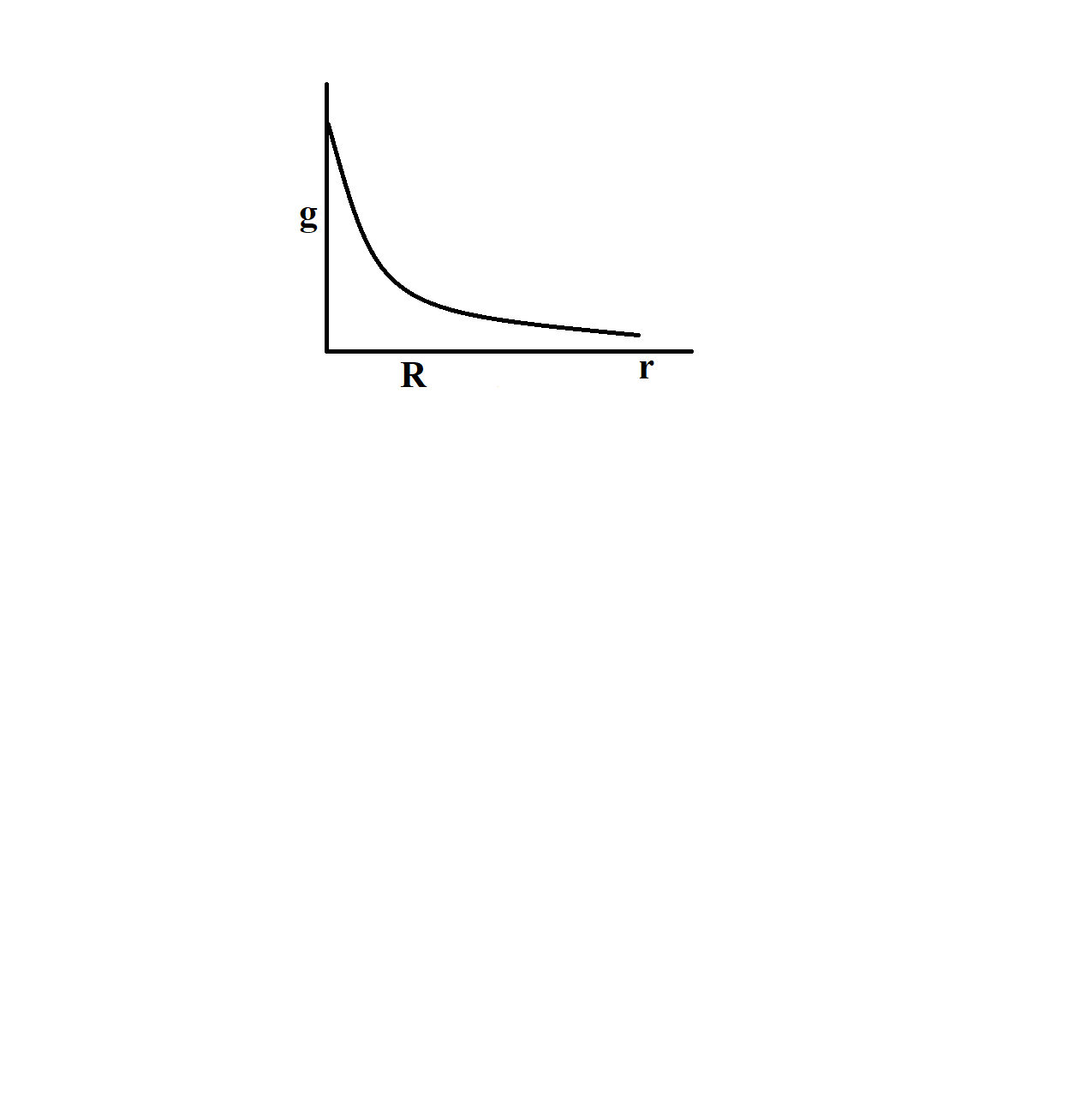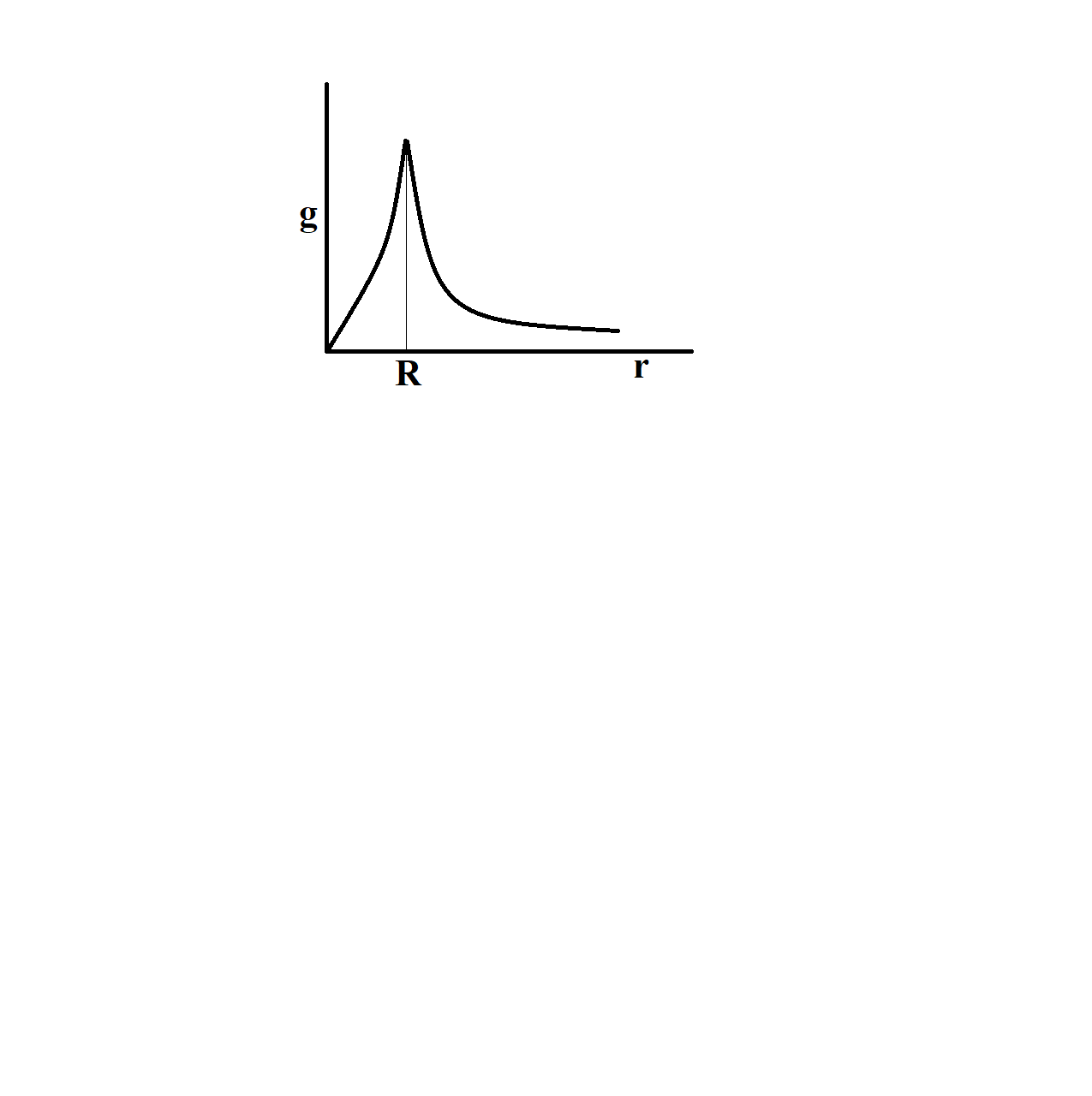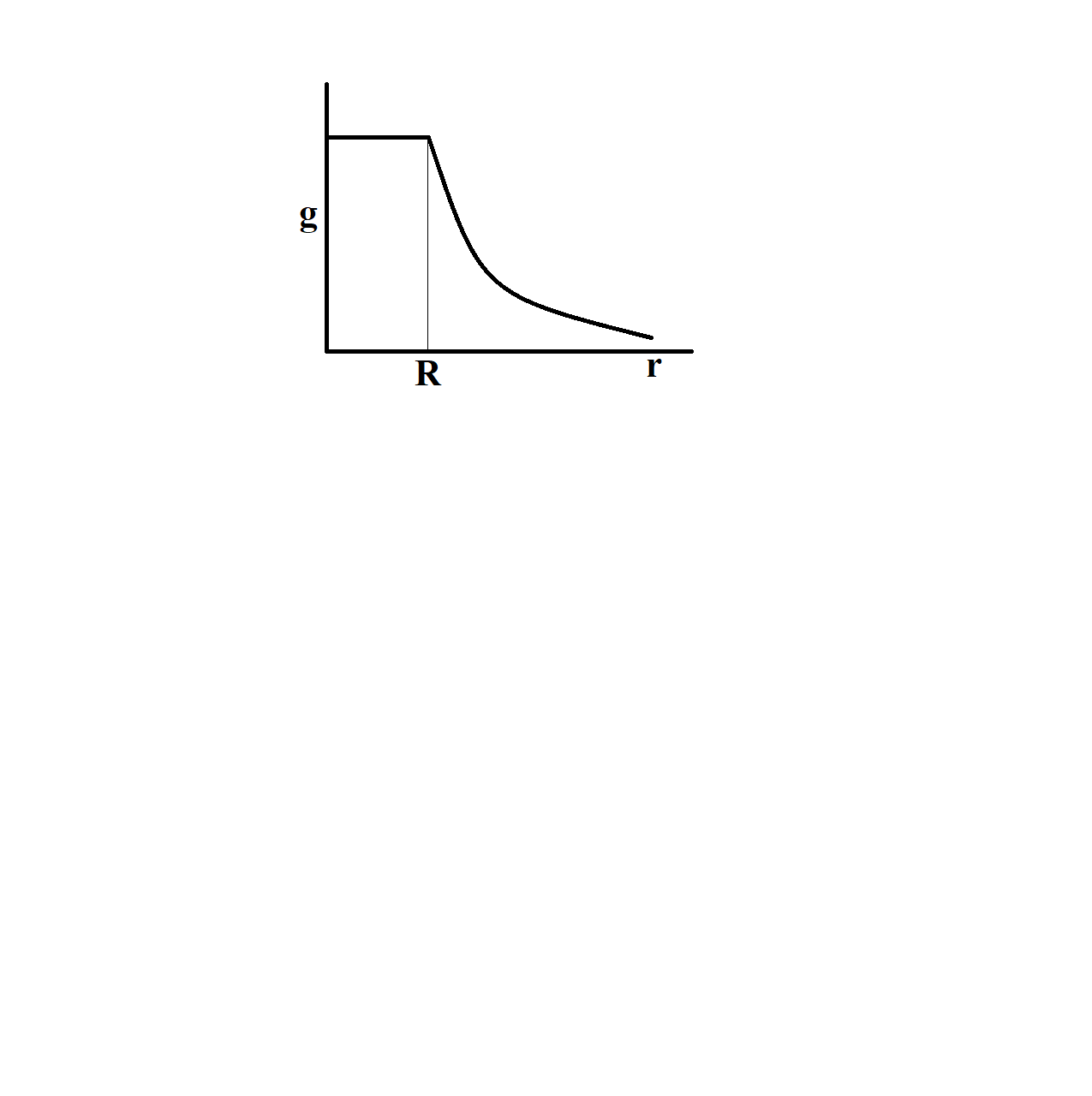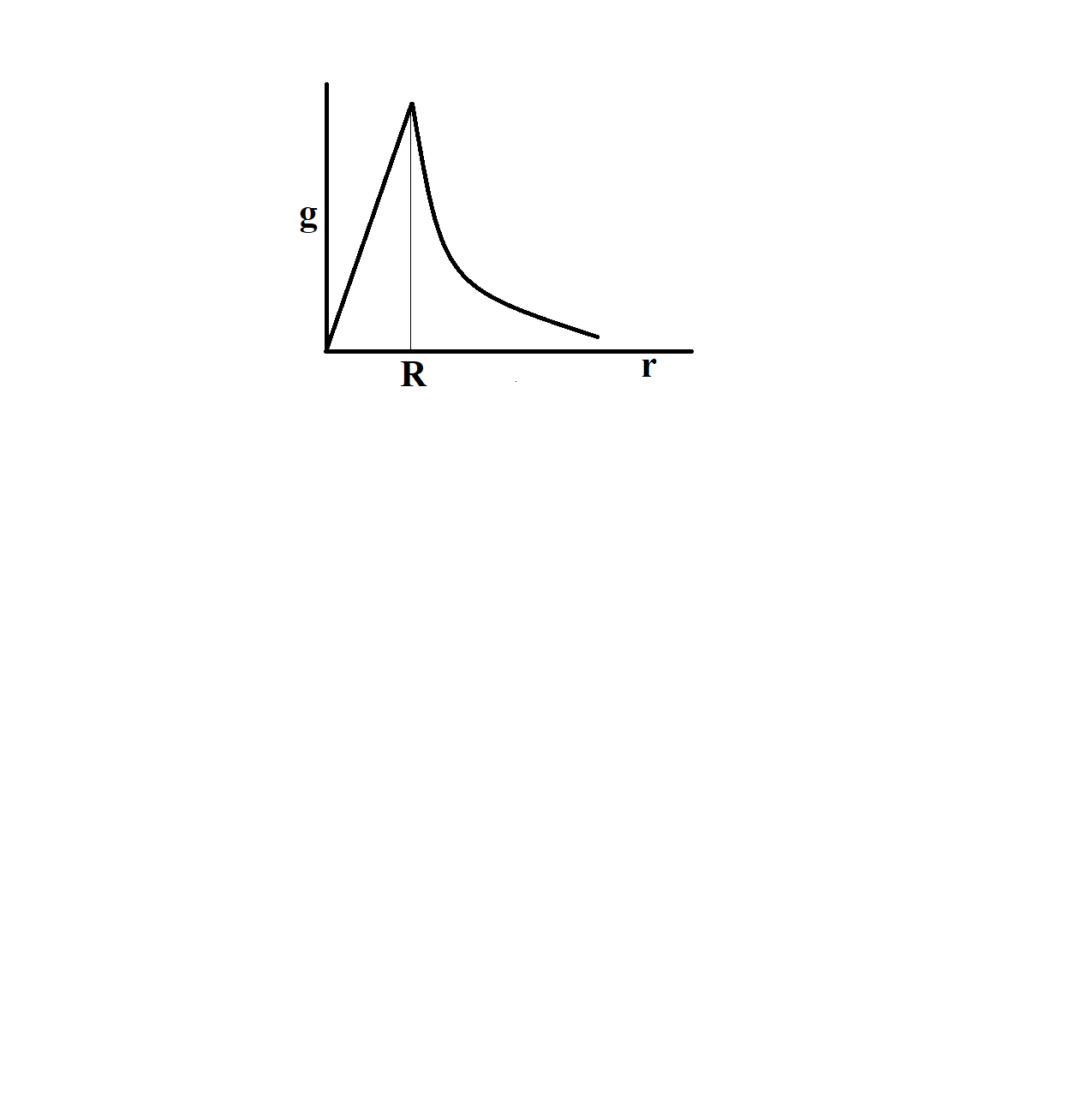QuestionAnswers

# The dependence of acceleration due to gravity $g$ on the distance $r$ from the centre of the Earth, assumed to be a sphere of radius $R$ of uniform density is as shown in figures below. The correct figure isHint: We will consider the variation of value of acceleration due to gravity with the distance from the centre of the earth. Two cases can arise, one for distance from the centre is smaller than the radius of the earth and the other case is distance from the centre is larger than the radius for earth.
Formula used-
For $R>r$ , $g'\propto r$
For $r>R$ , $g'\propto \dfrac{1}{{{r}^{2}}}$

Gravitational acceleration, or the acceleration due to gravity, is defined as the free fall acceleration of a body in vacuum, without any drag force. The acceleration due to gravity is the acceleration gained by a body because of gravitational force. Its SI unit is $m{{s}^{-2}}$ . Gravitational acceleration is a vector quantity, meaning it has both magnitude and direction.
The value of gravitational acceleration varies with altitude (height), depth, shape of earth and rotation of earth about its own axis.
The acceleration due to gravity at a depth $d$ below the surface of earth is given by,
\begin{align} & g'=\dfrac{GM}{{{R}^{2}}}\left( 1-\dfrac{d}{R} \right) \\ & g'=g\left( 1-\dfrac{d}{R} \right) \\ \end{align}
At the centre of Earth, $d=R$
$g'=0$
At the centre of earth, acceleration due to gravity is zero.
The acceleration due to gravity $g$ varies with distance $r$ , where $r>R$ , is given by,
\begin{align} & g'=\dfrac{g}{{{\left( 1+\dfrac{h}{R} \right)}^{2}}} \\ & g'=\dfrac{g{{R}^{2}}}{{{r}^{2}}} \\ & g'\propto \dfrac{1}{{{r}^{2}}} \\ \end{align}
Here, $R+h=r$
For, r \begin{align} & g'=g\left( 1-\dfrac{d}{R} \right) \\ & g'=\dfrac{gr}{R} \\ & g'\propto r \\ \end{align} Here, R-d=r $Thus, for$ r>R $, we have$ g'\propto \dfrac{1}{{{r}^{2}}} $And for For$ R>r $, we have$ g'\propto r \$
Hence, the correct option is D.

Note:
At a given place, for a fixed value of distance from the centre of earth, the value of acceleration due to gravity is constant. But the value of gravitational acceleration changes from one place to another. For an object placed at some height, the value of gravitational acceleration is less as compared to that placed on the surface of earth. As the depth increases, the value of gravitational acceleration falls.

View Notes
Electric Field Due to Point ChargeDerivation of Centripetal AccelerationAcceleration Time GraphGravity FormulaAverage Acceleration FormulaAcceleration FormulasAngular AccelerationWork Done by Gravity FormulaValue of gCBSE Class 11 Physics Law of Motion FormulasImportant Questions for CBSE Class 11 Physics Chapter 11 - Thermal Properties of MatterImportant Questions for CBSE Class 11 PhysicsImportant Questions for CBSE Class 11 Physics Chapter 5 - Law of MotionImportant Questions for CBSE Class 11 Physics Chapter 9 - Mechanical Properties of SolidsImportant Questions for CBSE Class 11 Physics Chapter 7 - Systems of Particles and Rotational MotionImportant Questions for CBSE Class 12 Physics Chapter 11 - Dual Nature of Radiation and MatterImportant Questions for CBSE Class 11 Physics Chapter 10 - Mechanical Properties of FluidsImportant Questions for CBSE Class 11 Accountancy Chapter 1 - Introduction To AccountingImportant Questions for CBSE Class 11 Physics Chapter 12 - ThermodynamicsCBSE Class 7 Science Weather, Climate and Adaptations of Animals to Climate WorksheetsCBSE Class 12 Physics Question Paper 2020Previous Year Question Paper for CBSE Class 12 PhysicsPrevious Year Question Paper for CBSE Class 12 Physics - 2015Previous Year Question Paper for CBSE Class 12 Physics - 2018Previous Year Question Paper for CBSE Class 12 Physics - 2014Previous Year Question Paper for CBSE Class 12 Physics - 2013Previous Year Question Paper for CBSE Class 12 Physics - 2019Previous Year Physics Question Paper for CBSE Class 12 - 2017Physics Question Paper for CBSE Class 12 - 2016 Set 1 CPrevious Year Question Paper for CBSE Class 12 Physics - 2016 Set 1 STextbooks Solutions for CBSE & ICSE Board of Class 6 to 12 Maths & ScienceClass 11 Physics NCERT solutionsNCERT Solutions Class 11 English Woven Words Poem Chapter 2 Let Me Not to the Marriage of True MindsNCERT Exemplar for Class 11 Physics Chapter 11 - Thermal Properties of Matter (Book Solutions)NCERT Solutions for Class 12 English Vistas Chapter 3 - Journey to the end of the EarthNCERT Solutions for Class 11 Physics Chapter 11 Thermal Properties of Matter in HindiLakhmir Singh Physics Class 9 Solutions Chapter 2 - Force and laws of motionNCERT Solutions for Class 11 Physics Chapter 11NCERT Solutions for Class 11 PhysicsNCERT Solutions Class 11 English Woven Words Poem Chapter 11 Ode to a Nightingale